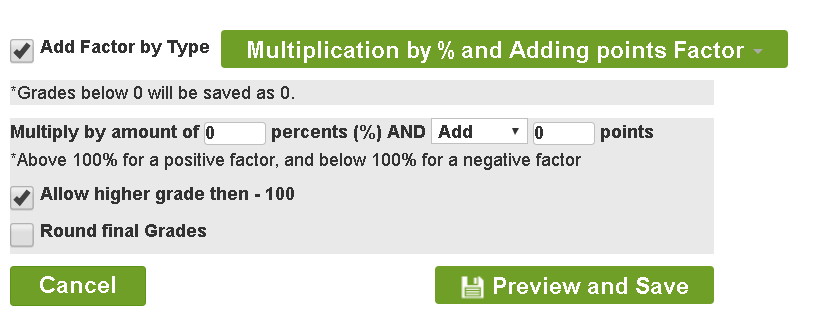General Factor

Add or subtract the same score for all students2. 100% refers to the maximum score defined in the original set of grades. Check in order to enable a higher final score, or, in the event of a reduction in order to enable a score reduction for the candidate who received 100%
3. Check in order to round up a final score for an integer (the rounding will be according to the rounding rules used by the institution)

Please note: No score is less than 0

Relative (percents) Factor1. Please note: enter a number greater than 100% for a positive factor and less than 100% for a negative one
2. Checking the box will allow, if a negative factor is chosen, to reduce the score even for students who received originally 100% of the score

Multiplication by % and Adding points FactorUsing the formula, for example: 75*0.85+17=80.75

Average and Standard Deviation Factor

Determining the desired average and standard deviation, the system will calculate the grades again according to the highlighted formula:Please note: this factor may reduce high scores

Square root then multiplication by 10 Factor

Left-skewed distributionFormula for calculation: X*10